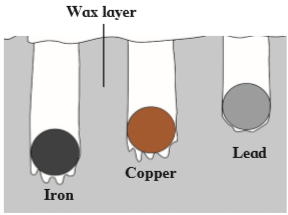SSC BOARD PAPERS IMPORTANT TOPICS COVERED FOR BOARD EXAM 2024

### Question 3. What is meant by specific heat capacity? How will you prove experimentally that different substances have different specific heat capacities?

Question 3.

What is meant by specific heat capacity? How will you prove experimentally that different substances have different specific heat capacities?

The amount of heat energy required to raise the temperature of a unit mass of an object by 1° C is called the specific heat capacity of that object.

To prove experimentally:(i) Take three spheres of iron, copper and lead of equal masses.

(ii) Now put all the three spheres in boiling water in the beaker for some time.

(iii) Now, take them all out of the water, we will notice that all the spheres will be at temperature 100° C.

(iv) Now, put them immediately on the thick slab of wax.

(v) Note the depth of each sphere into the wax.

(vi) The sphere which has absorbed more heat from water will give more heat to wax.

(vii) More wax will thus melt, and deeper the sphere will go in the wax.

(viii) We will notice, iron goes deepest, lead sphere goes the least and copper sphere goes to intermediate depth.

(ix) This shows that for equal rise in temperature, the three spheres have absorbed different amounts of heat.

(x) This means that the property which determines the amount of heat absorbed by a sphere is different for the three spheres.

(xi) This property is called the specific heat capacity.